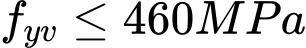Odkaz jsme Vám odeslali na email.

Nepodařilo se nám odeslat odkaz na váš email. Zkontrolujte, prosím, Váš email.

# Online nápověda

Stromeček
Nastavení
Produkt:
Program:
Jazyk:

## Verification of rectangular RC cross-section

The cross-section is rectangular, unilaterally reinforced and loaded by the bending moment and normal compression force. The program verifies a reinforced concrete section using the method of limit deformation. The maximum allowable strain of concrete in compression is 0,002 - 0,0035. Compression reinforcement is not taken into account. Minimum eccentricity is applied: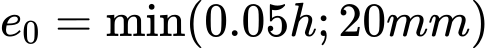The computed degree of reinforcement is checked using the following expressions: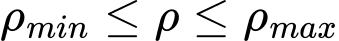where: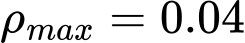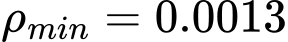- for  fy = 460 N/mm2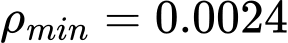- for  fy = 250 N/mm2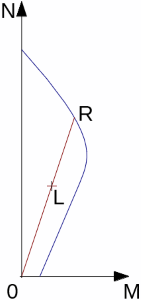Interaction diagram N-M

Usage ratio of concrete cross-section subject to the combination of bending moment and normal force is determined as |0L| / |0R|. Where L is load and R is strength with prescribed excentricity.

## Bending without normal force

The cross-section is rectangular, reinforced on one side and loaded by the bending moment Mu.

The permissible moment for a given area of reinforcements As reads: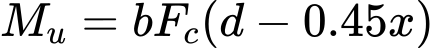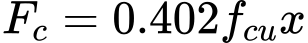The program further checks whether the location of neutral axis x is less than the limit location of neutral axis xmax given by: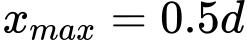## Shear

First, the program computes the ultimate shear strength of concrete Vc.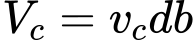where:The νc values are for fcu above 25 N/mm2 multiplied by  (fcu / 25)1/3

If the ultimate shear strength of concrete is exceeded, the ultimate shear strength Vmax is checked.Next, the necessary reinforcement area is given by: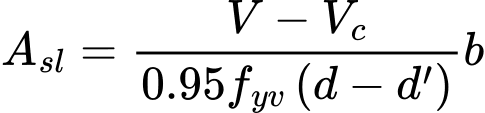where: